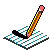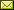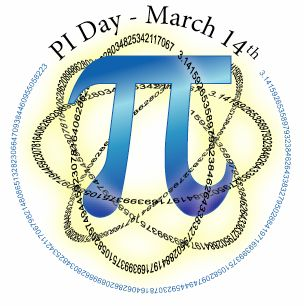PHYSICS@HORTONPhysics12

Physics 11

Math 11

NS Exams

Curriculum

Tips

Tutorials

McGraw Hill

Resources

Need it?

News

Enrichment

GiancoliMATH11

March 2012 Notes

 March 1 From Graphs to Equations More Applications Practice: Parameters & Equations of Sinusoidal Functions (answers) Practice: Understanding Sinusoidal Functions (answers) Notes March 5 CONCEPT CHECK: Interpreting Graphs Applications with Equations Practice: Sinusoidal Models (with Equations) (answers) Notes March 7 Other Sinusoidal Functions Practice: Identifying Sinusoidal Graphs (answers) Group Work: Interpreting Sinusoidal Situations Notes March 9 Work on Group Work March 14March 20 Sinusoidal Equations & what they tell us Group Work: Sinusoidal Functions Check-up Notes March 22 Start working on test outline & review (answers) March 26 Soving Sinusoidal Equations Graphically Practice: Solving trig equations graphically (all solutions) (answers) Notes March 28 Solving Sinusoidal Equations Algebraically Concept Check: Sinusoidal Applications with equation given Practice: Solving Trigonometric Equations Notes March 30 Solving Sinusoidal Equations Algebraically Practice: Sinusoidal Functions (answers) Groupwork: Sinusoidal models (given equation) Notes